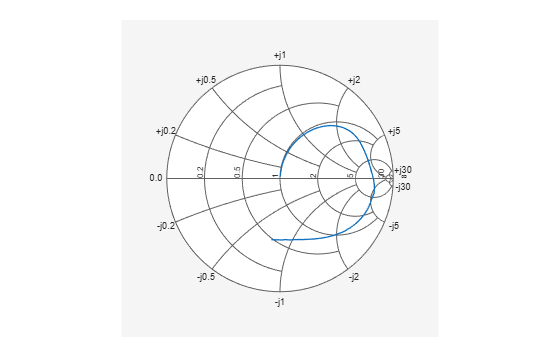# Operations with RF Data Objects

This example shows you how to manipulate RF data directly using `rfdata` objects. First, you create an `rfdata.data` object by reading in the S-parameters of a two-port passive network stored in the Touchstone® format data file, `passive.s2p`. Next, you create a circuit object, `rfckt.amplifier`, and you update the properties of this object using three data objects.

Use the `read` method of the `rfdata.data` object to read the Touchstone data file `passive.s2p`. The parameters in this data file are the 50-Ohm S-parameters of a 2-port passive network at frequencies ranging from 315 kHz to 6.0 GHz.

```data = rfdata.data; data = read(data,'passive.s2p')```
```data = rfdata.data with properties: Freq: [202x1 double] S_Parameters: [2x2x202 double] GroupDelay: [202x1 double] NF: [202x1 double] OIP3: [202x1 double] Z0: 50.0000 + 0.0000i ZS: 50.0000 + 0.0000i ZL: 50.0000 + 0.0000i IntpType: 'Linear' Name: 'Data object' ```

Use the `extract` method of the `rfdata.data` object to get other network parameters. For example, here are the frequencies, 75-Ohm S-parameters, and Y-parameters which are converted from the original 50-Ohm S-parameters in `passive.s2p` data file.

```[s_params,freq] = extract(data,'S_PARAMETERS',75); y_params = extract(data,'Y_PARAMETERS');```

Use the RF utility function, `smithplot` to plot the 75-Ohm S11 on a Smith chart.

```s11 = s_params(1,1,:); figure smithplot(freq, s11(:))```Here are the four 75-Ohm S-parameters and four Y-parameters at 6.0 GHz, the last frequency.

`f = freq(end)`
```f = 6.0000e+09 ```
`s = s_params(:,:,end)`
```s = 2×2 complex -0.0764 - 0.5401i 0.6087 - 0.3018i 0.6094 - 0.3020i -0.1211 - 0.5223i ```
`y = y_params(:,:,end)`
```y = 2×2 complex 0.0210 + 0.0252i -0.0215 - 0.0184i -0.0215 - 0.0185i 0.0224 + 0.0266i ```

### Create RF Data Objects for Amplifier with Your Own Data

In this example, you create a circuit object, `rfckt.amplifier`. Then you create three data objects and use them to update the properties of the circuit object.

The `rfckt.amplifier` object has properties for network parameters, noise data and nonlinear data:

By default, these properties of `rfckt.amplifier` contain data from the `default.amp` data file. `NetworkData` is an `rfdata.network` object that contains 50-Ohm 2-port S-Parameters at 191 frequencies ranging from 1.0 GHz to 2.9 GHz. `NoiseData` is an `rfdata.noise` object that contains spot noise data at 9 frequencies ranging from 1.9 GHz to 2.48 GHz. The `NonlinearData` parameter is an `rfdata.power` object that contains `Pin/Pout` data at 2.1 GHz.

`amp = rfckt.amplifier`
```amp = rfckt.amplifier with properties: NoiseData: [1x1 rfdata.noise] NonlinearData: [1x1 rfdata.power] IntpType: 'Linear' NetworkData: [1x1 rfdata.network] nPort: 2 AnalyzedResult: [1x1 rfdata.data] Name: 'Amplifier' ```

Use the following code to create an `rfdata.network` object that contains 2-port Y-parameters of an amplifier at 2.08 GHz, 2.10 GHz and 2.15 GHz. Later in this example, you use this data object to update the `NetworkData` property of the amplifier object.

```f = [2.08 2.10 2.15] * 1.0e9; y(:,:,1) = [-.0090-.0104i, .0013+.0018i; -.2947+.2961i, .0252+.0075i]; y(:,:,2) = [-.0086-.0047i, .0014+.0019i; -.3047+.3083i, .0251+.0086i]; y(:,:,3) = [-.0051+.0130i, .0017+.0020i; -.3335+.3861i, .0282+.0110i]; netdata = rfdata.network('Type','Y_PARAMETERS','Freq',f,'Data',y)```
```netdata = rfdata.network with properties: Type: 'Y_PARAMETERS' Freq: [3x1 double] Data: [2x2x3 double] Z0: 50.0000 + 0.0000i Name: 'Network parameters' ```

Use the following code to create an `rfdata.nf` object that contains noise figures of the amplifier, in dB, at seven frequencies ranging from 1.93 GHz to 2.40 GHz. Later in this example, you use this data object to update the `NoiseData` property of the amplifier object.

```f = [1.93 2.06 2.08 2.10 2.15 2.3 2.4] * 1.0e+009; nf = [12.4521 13.2466 13.6853 14.0612 13.4111 12.9499 13.3244]; nfdata = rfdata.nf('Freq',f,'Data',nf)```
```nfdata = rfdata.nf with properties: Freq: [7x1 double] Data: [7x1 double] Name: 'Noise figure' ```

Use the following code to create an `rfdata.ip3` object that contains the output third-order intercept points of the amplifier, which is 8.45 watts at 2.1 GHz. Later in this example, you use this data object to update the `NonlinearData` property of the amplifier object.

`ip3data = rfdata.ip3('Type','OIP3','Freq',2.1e9,'Data',8.45)`
```ip3data = rfdata.ip3 with properties: Type: 'OIP3' Freq: 2.1000e+09 Data: 8.4500 Name: '3rd order intercept' ```

Use the following code to update the properties of the amplifier object with three data objects you created in the previous steps. To get a good amplifier object, the data in these data objects must be accurate. These data could be obtained from RF measurements, or circuit simulation using other tools.

```amp.NetworkData = netdata; amp.NoiseData = nfdata; amp.NonlinearData = ip3data```
```amp = rfckt.amplifier with properties: NoiseData: [1x1 rfdata.nf] NonlinearData: [1x1 rfdata.ip3] IntpType: 'Linear' NetworkData: [1x1 rfdata.network] nPort: 2 AnalyzedResult: [1x1 rfdata.data] Name: 'Amplifier' ```